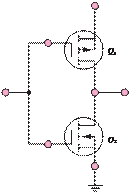### Create an Account

Already have account?

### Forgot Your Password ?

Home / Questions / Draw the schematic diagram of a two input CMOS OR gate 11 30 Draw the schematic di...

# Draw the schematic diagram of a two input CMOS OR gate 11 30 Draw the schematic diagram of a two input CMOS AND gate 11 31 Draw the schematic diagram of a two input CMOS NOR gate

Draw the schematic diagram of a two-input

CMOS OR gate.

11.30      Draw the schematic diagram of a two-input CMOS AND gate.

11.31      Draw the schematic diagram of a two-input CMOS NOR gate.

11.32      Draw the schematic diagram of a two-input CMOS NAND gate.

11.33      Show that the circuit of Figure P11.33 functions as a logic inverter.

V

_

VDDI

Jun 18 2020 View more View Less

#### Answer (Solved)Subscribe To Get Solution